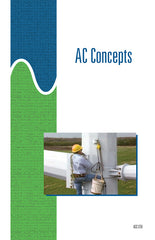#### Get the Study Guide for this videoFollow the video session-by-session with this handy book. Color illustrations throughout. \$15

Instructor Guide  available

# AC Concepts - DVD

### \$ 145.00

##### Summary

This 32-minute DVD examines various aspects of AC power. It shows the relationships between AC generator operation, and sine wave and phasor representations of AC voltage and current. It introduces the effects of inductance and capacitance in an AC circuit and how these effects can be illustrated using sine waves and phasor diagrams. It also covers the relationships between active power, reactive power, and apparent power and introduces the concept of power factor.

Note: This DVD is similar to AC Fundamentals Review. It is slanted toward how alternating current is metered, while AC Fundamentals Review has more general coverage. Since alternating current is not an easy concept to grasp, watching both DVDs is often helpful.

##### Training Sessions

Magnitude, Direction, and Time – 10 minutes
Describes how a basic AC generator works to produce voltage and current. Shows how the magnitude and direction of AC voltage and (in-phase) current can be plotted against time using sine waves, and how current can be shown for a given point in time.

Inductance – 6 minutes
Defines inductance and inductive reactance. Describes how an inductor works to oppose changes in AC current. Uses sine waves and phasors to explains the effects of inductance and capacitance on AC voltage and current.

Capacitance – 6 minutes
Defines capacitance and capacitive reactance. Explains how a capacitor works to oppose changes in AC voltage. Shows the effects of capacitance on AC voltage and current using sine waves and phasors.

AC Power – 10 minutes
Differentiates between active power, reactive power, and apparent power. Explains how power factor is used in calculating active power in AC circuits.

Item Code: ACC-DVD